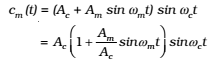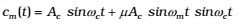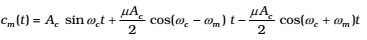## Amplitude Modulation

In amplitude modulation, the amplitude of the carrier is varied in accordance with the information signal.

AM can be represented by expression

Let c(t) = Ac sinωct represent carrier wave and m(t) = Am sint represent the message or the modulating signal where m = 2fm is the angular frequency of the message signal. The modulated signal cm (t) can be written asHere μ = Am/Ac is the modulation index; in practice, μ is kept ≤ 1 to avoid distortion.
Using the trigonometric relation sinA sinB = ½ (cos(A – B) – cos (A + B), we can write cm(t)Here ωc – ωm and ωc + ωm are respectively called the lower side and upper side frequencies. The modulated signal now consists of the carrier wave of frequency ωc plus two sinusoidal waves each with a frequency slightly different from, known as side bands. The frequency spectrum of the amplitude modulated signal is shown in Fig.Production of AM Wave: One of the simplest methods is shown below by block diagram.